# When analyzing recursive algorithms, which of the following best describes initial conditions? Initial conditions express the...

When analyzing recursive algorithms, which of the following best describes initial conditions?

 Initial conditions express the complexity of an algorithm
 Initial conditions express the number of times the basic operation executes when the base case is reached.
 Initial conditions express the number of times the basic operation runs during the first execution of the function.
 Initial conditions represent the condition for the base case of a recursive function

```When analyzing recursive algorithms, Initial conditions represent the condition for the base case of a recursive function
```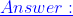Option 4

#### Earn Coin

Coins can be redeemed for fabulous gifts.

Similar Homework Help Questions
• ### ld ts biovs Part II: Analysis of recursive algorithms is somewhat different from that of non-recursive...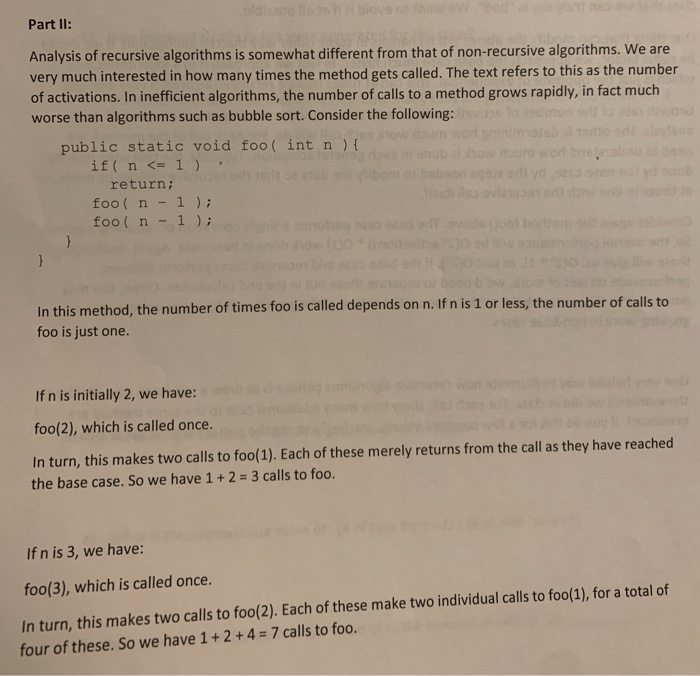ld ts biovs Part II: Analysis of recursive algorithms is somewhat different from that of non-recursive algorithms. We are very much interested in how many times the method gets called. The text refers to this as the number of activations. In inefficient algorithms, the number of calls to a method grows rapidly, in fact much worse than algorithms such as bubble sort. Consider the following: public static void foo ( int n ) { if n <=1 ow ura wor...

• ### Which of the following statements best describes when an asset should be replaced? I - The...

Which of the following statements best describes when an asset should be replaced? I - The defender should be used until its annual cost of operation exceeds the annual cost of using the challenger. II - The challenger should replace the defender at the end of the defender's lifespan. III - The challenger should replace the defender whenever the challenger's ESL is reached.

• ### [Recursive Cost] [ALGORITHM] Improving Efficiency PLEASE explain in DETAIL the following question in detail. The algorithm...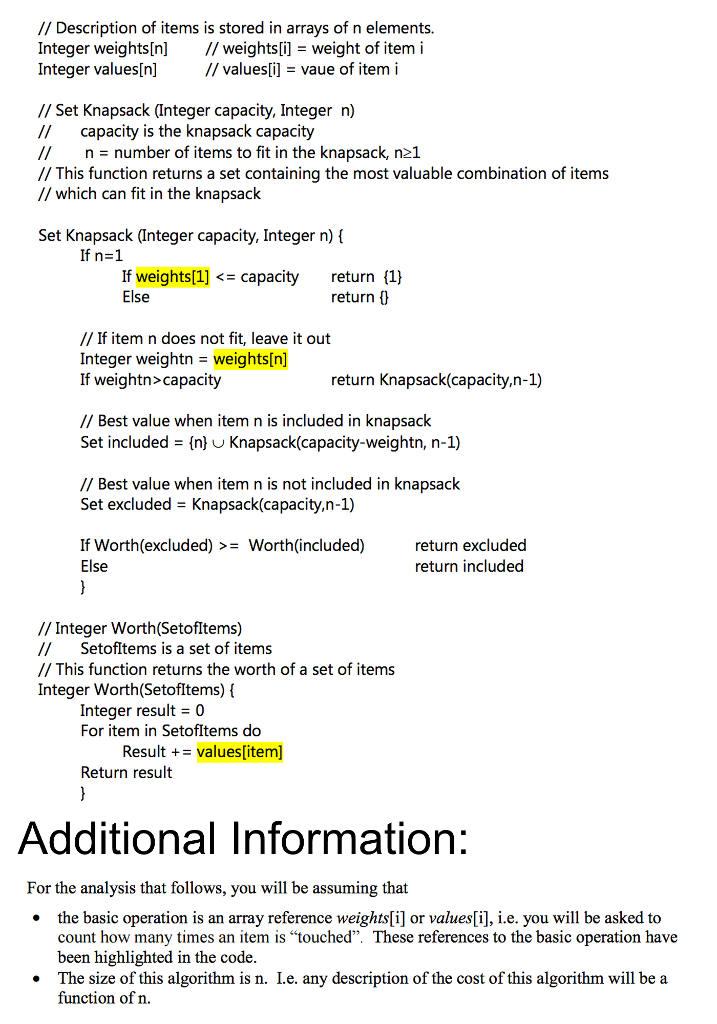[Recursive Cost] [ALGORITHM] Improving Efficiency PLEASE explain in DETAIL the following question in detail. The algorithm is also given below. Thank You! 1.a) Define recursively the worst case cost Kn of the Knapsack function for n items. Remember that you need to provide both the base case and the recurrence relation. Also do not forget to include the cost of the function Worth in your cost. Justify your answer (i.e. explain what each component of the formula represents). [5points] 1.b)  Use...

• ### Which of the following statements best describes when an asset should be replaced? 1 - The...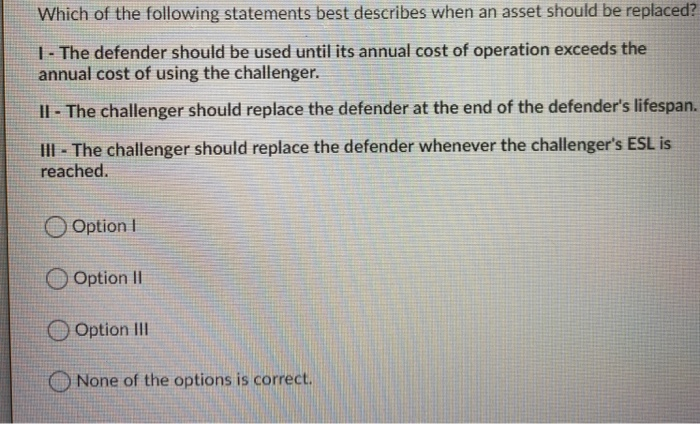Which of the following statements best describes when an asset should be replaced? 1 - The defender should be used until its annual cost of operation exceeds the annual cost of using the challenger. 11 - The challenger should replace the defender at the end of the defender's lifespan. III - The challenger should replace the defender whenever the challenger's ESL is reached Option 1 Option 11 Option III None of the options is correct.

• ### Question 4 (10 marks) When analysing the complexity of algorithms, there are three main approaches: worst case, best ca...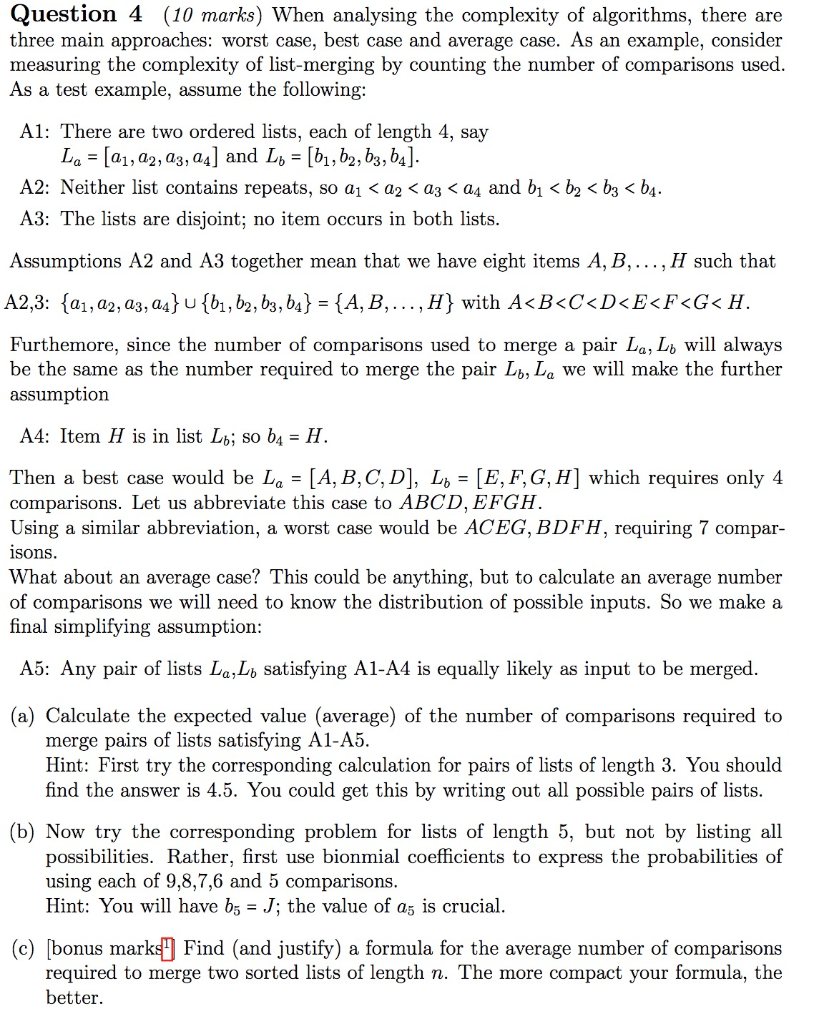Question 4 (10 marks) When analysing the complexity of algorithms, there are three main approaches: worst case, best case and average case. As an example, consider measuring the complexity of list-merging by counting the number of comparisons used As a test example, assume the following A1: There are two ordered lists, each of length 4, say A2: Neither list contains repeats, so a! < a2 < аз < a4 and bl <b2 < b3 < b4 A3: The lists are...

• ### For each problems segment given below, do the following: Create an algorithm to solve the problem...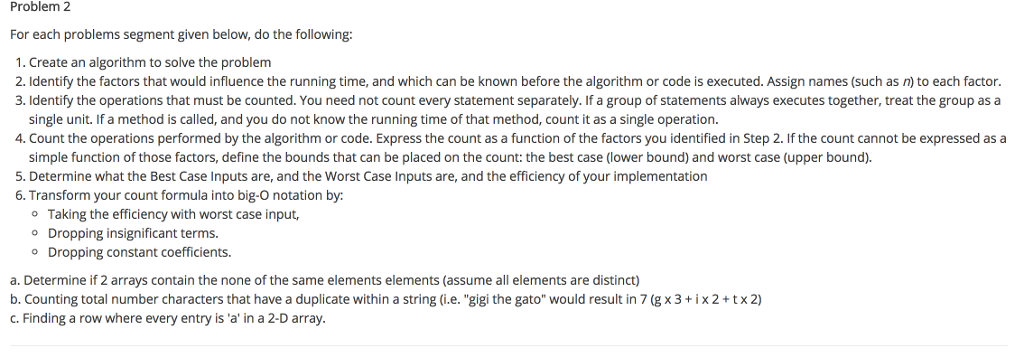For each problems segment given below, do the following: Create an algorithm to solve the problem Identify the factors that would influence the running time, and which can be known before the algorithm or code is executed. Assign names (such as n) to each factor. Identify the operations that must be counted. You need not count every statement separately. If a group of statements always executes together, treat the group as a single unit. If a method is called, and...

• ### Practical 5: Write a program that implements several sorting algorithms, and use it to demonstrate the comparative perfo...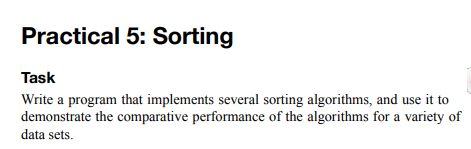Practical 5: Write a program that implements several sorting algorithms, and use it to demonstrate the comparative performance of the algorithms for a variety of data sets. Need Help With this Sorting Algorithm task for C++ Base Code for sorting.cpp is given. The header file is not included in this. Help would be much appreciated as I have not started on this due to personal reasons #include <cstdlib> #include <iostream> #include <getopt.h> using namespace std; long compares; // for counting...

• ### Help needed with multiple choice Qs

Question1:You are using a language that does not support recursion. What data structure would you use to traverse a binary search tree in order?1. Set Next(newNode) to location2. Set Back(location) to newNode3. Set Next(Back(location)) to newNode 4. Set Back(newNode) to Back(location)a) queueb) listc) stackd) can't traverse a tree in order without recursionQuestion 2int z(int k, int n){if (n == k)return kelse{if (n > k)return z(k, n-k);else return z(k-n, n);}}Based on the function defined above, what is the value of z(6,8)?a)...

• ### Which of the following statements best describes the difference between a benign and malignant tumor?_____ (type...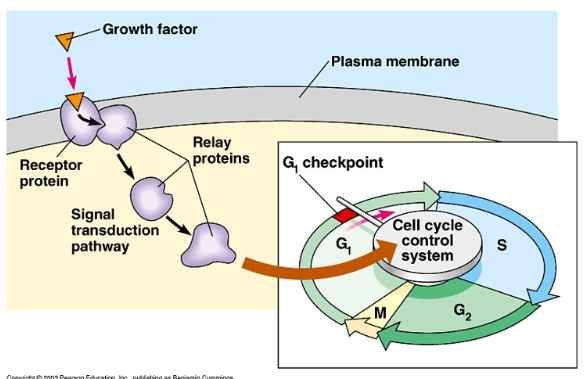Which of the following statements best describes the difference between a benign and malignant tumor?_____ (type 1, 2, or 3 in the blank) 1. benign tumors rarely interfere with organ function but malignant tumors often impair and spread to other organs. 2. benign tumors are produced from unregulated cell growth but malignant tumors are regulated 3. benign tumors metastasize but malignant tumors do not Which of the following statements best describes metastasis? ____ 1. metastasis is unregulated growth of cells...

• ### python plz 2. Which of the following most appropriately describes a function? A. Play one-game uses...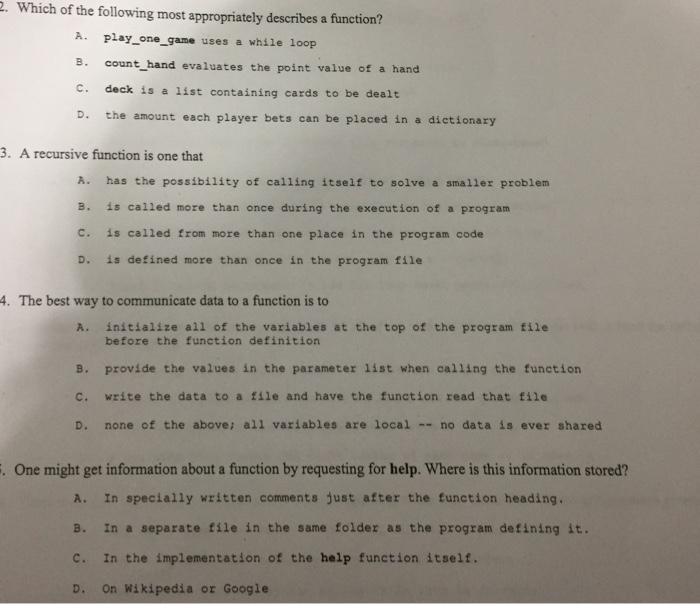python plz 2. Which of the following most appropriately describes a function? A. Play one-game uses a whie 1oop B. count hand evaluates the point value of a hand c. deck is a list containing cards to be dealt D. the amount each player bets can be placed in a dictionary 3. A recursive function is one that A. has the possibility of calling itself to solve a smaller problem B. is called more than once during the execution of...